• 采用matlab的gui技术生成的界面轨道运行程序
• 运行MATLAB运行MATLAB软件，打开后界面如下图所示：此为MATLAB软件界面，主要分为上图中6个部分：菜单栏/工具栏工作目录工作目录中文件目录目录窗口工作区命令历史记录3.菜单栏/工具栏介绍对于菜单栏/工具...


1.首先打开MATLAB软件：MATLAB在安装中如果没有选择在桌面生成快捷方式，则需要去安装目录下自己查找，具体路径如下图：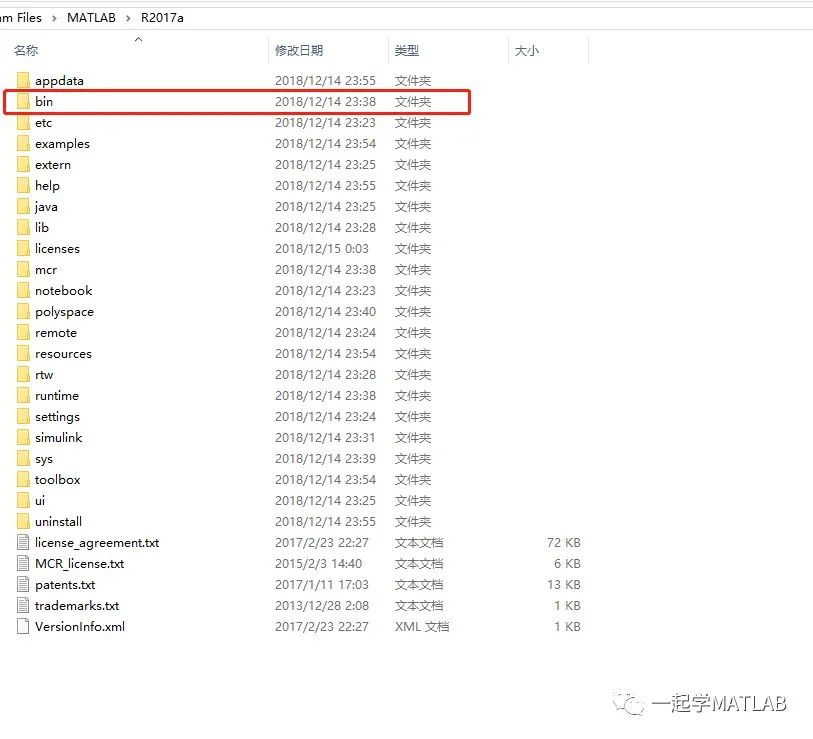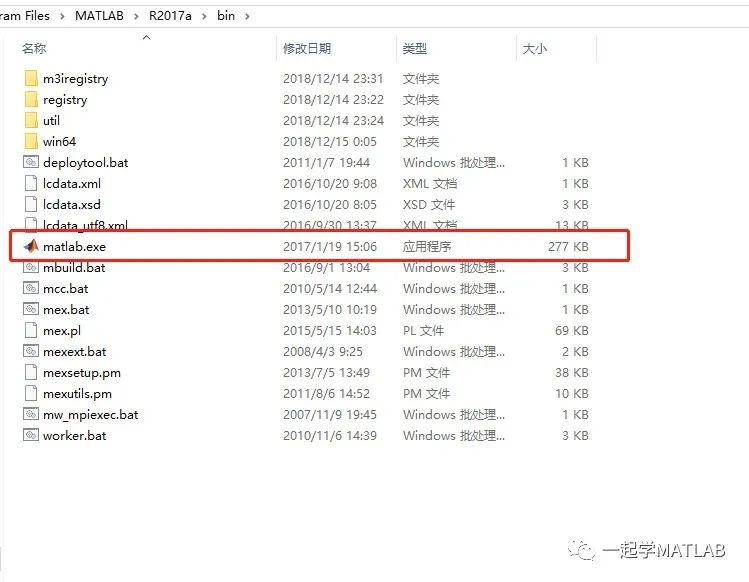2.运行MATLAB运行MATLAB软件，打开后界面如下图所示：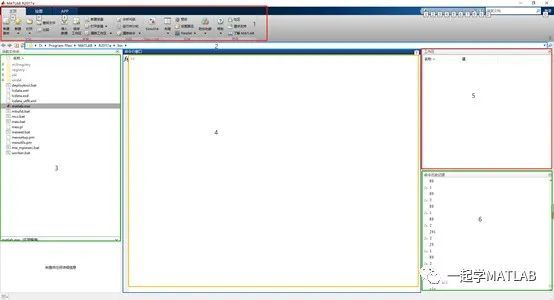此为MATLAB软件的主界面，主要分为上图中的6个部分：菜单栏/工具栏工作目录工作目录中的文件目录目录窗口工作区命令历史记录3.菜单栏/工具栏的介绍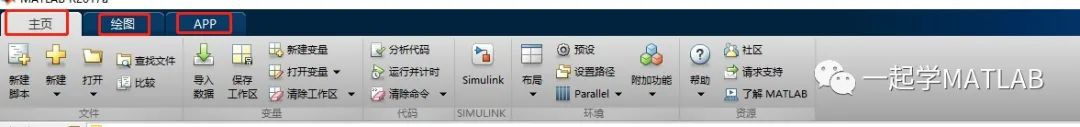对于菜单栏/工具栏来讲，主要有三个选项卡，如图中红色标记：主页绘图 和 APP。其中主页选项卡主要包含六大模块：文件变量，代码，Simulink，环境和资源模块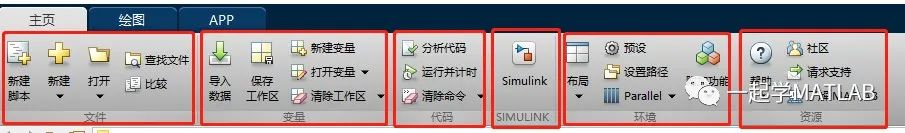其中新建脚本可以创建一个新的MATLAB脚本。新建下则有以下选项，可以选择新建：脚本，函数，示例，类，System Object(专用的MATLAB对象，专为实现和仿真输入随时间变化的动态系统而设计)，图形界面，APP工具箱及GUI界面，命令快捷方式等。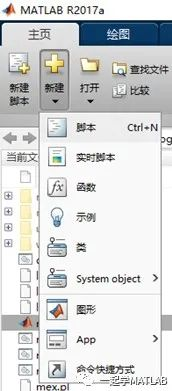变量进行操作的区域，主要包含导入数据、保存工作空间、打开变量、新建变量、清除工作区(清除变量和清除工作空间)的操作。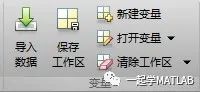代码区域是由分析代码(可以打开代码报告，里面有可能的报错信息，帮助编程者优化程序)、执行代码和计时、清除命令三大部分构成。Simulink区域可以打开Simulink的库。环境区域可以更改软件的一些默认设置，例如背景、默认路径等。资源区域可以搜索网上关于MATLAB的资源。工具栏的Plot是进行画图的快捷方式，可以绘制直方图、线图、饼图、等值图、三维图等不同种类的图形。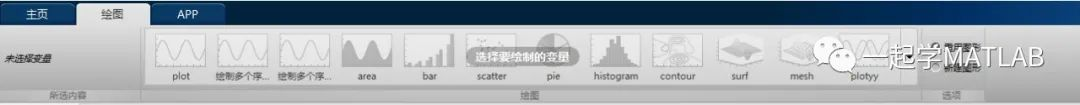最后的APPS一栏提供了不同种类的工具箱支持，方便用户进行快捷的操作。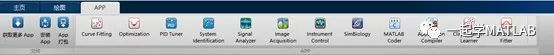4.文件路径及当前文件夹窗口介绍文件路径是当前文件夹的地址，在该区域可以实现文件路径的切换。当前文件夹是显示当前文件路径下所有文件的窗口，可以在此双击打开所需要的.m等不同格式的文件。也可以在此窗口对文件进行操作，实现复制，剪切，粘贴和删除操作，同时也可以双击此窗口的文件夹实现对当前文件路径的切换工作。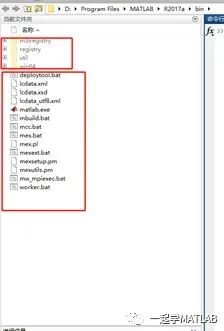5. 命令窗口介绍命令窗口是进行一系列命令输入的地方，当有指令输入并按下Enter键时，软件会自动执行该条指令，并执行出该命令的结果。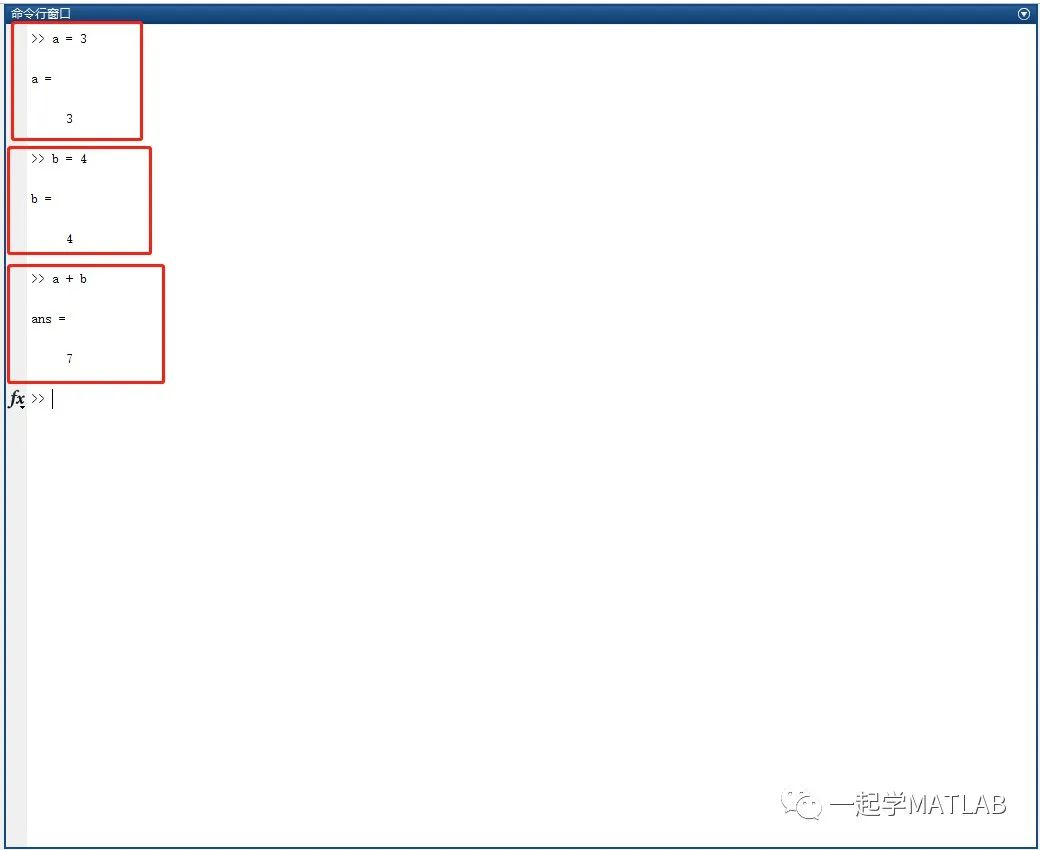如果不想每个命令后都显示运算结果，可以在代码结束后加一个“;”即效果则可为这样：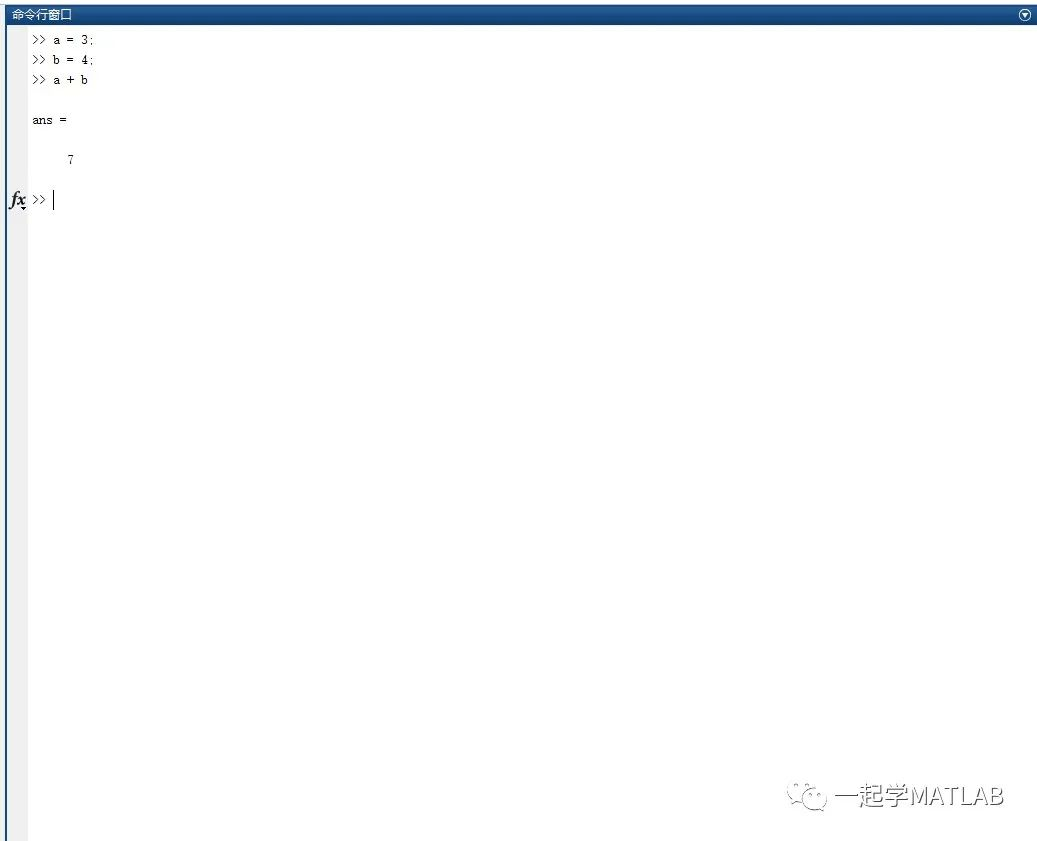6. 工作变量区介绍工作变量区域是存放所执行程序中涉及到的所有变量值的空间，可以在该区域双击某变量查看其具体的变量表示情况。如刚才命令所示，定义了两个变量 a 和 b 并同时进行了a+b运算生成了ans变量，结果显示在工作区中。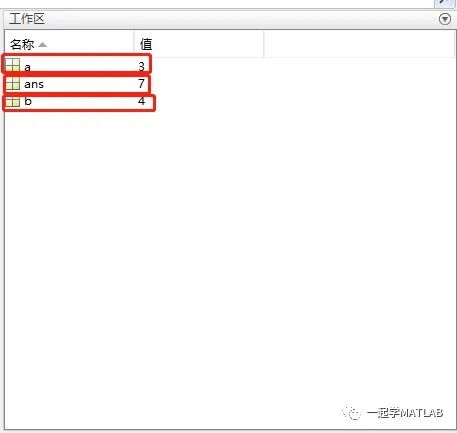7. 命令历史记录窗口介绍命令历史记录窗口主要是记录历史命令的窗口。在窗口中会以每天的方式进行记录，并且通过双击历史记录可以自动显示在命令行窗口内。同时按下方向键中向上和向下键进行调整选择历史命令。如果按下向上键则：刚才最后一条命令a+b自动显示在命令窗口中了。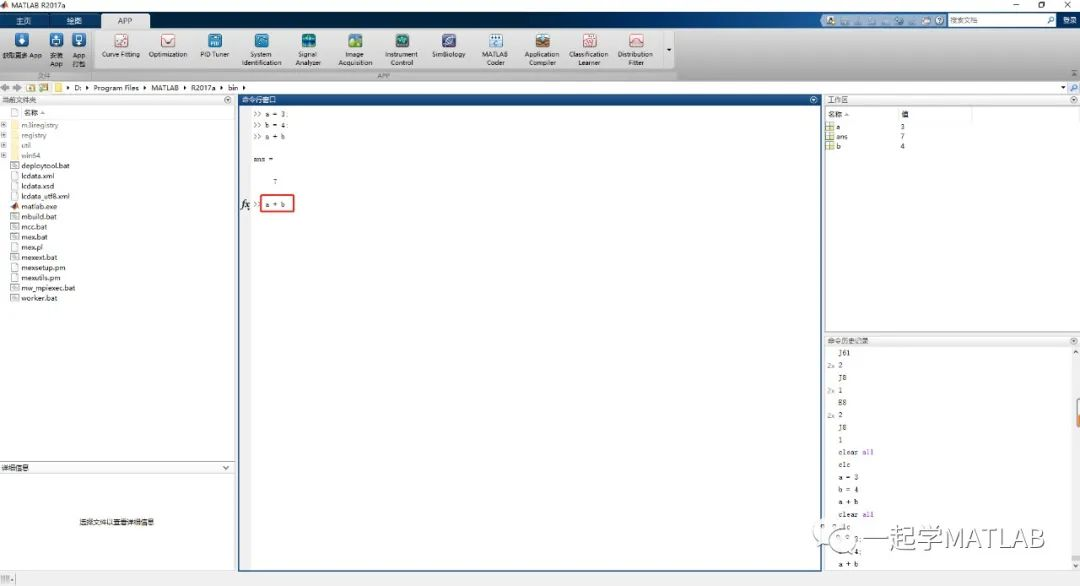以上为MATLAB软件的界面相关的介绍。后续会逐渐深入的讲解MATLAB的基本知识，带领小伙伴入门MATLAB。我会不定期进行更新相关内容，后续会再介绍MATLAB对图像处理的应用及OpenCV相关的知识。以及python和人工智能相关领域也会一点点介绍哦。如果对MATLAB感兴趣的小伙伴可以关注我的公众号哦。

展开全文• 本人已经测试过，没有错误，功能强大，基于Matlab的GUI界面设计，运行无误。
• 点击signal_generator.fig即可运行整个程序，代码在matlab R2018b上绝对完美运行，GUI纯小白建议预习再下，如果不会打开GUIDE，百度一下再打开，不要报了bug就说代码不能运行
• 今日做吸引域仿真，由于需要遍历100*100*100空间，需要时间比较长，发现程序没运行一段时间，就会出现Out of memory错误，而且出错部分在于截取figure内部图片部分。 开始时以为是因为申请数组太大...

今日做吸引域的仿真，由于需要遍历100*100*100的空间，需要的时间比较长，发现程序没运行一段时间，就会出现Out of memory的错误，而且出错的部分在于截取figure内部图片的部分。  开始时以为是因为申请的数组太大的原因，即开始时申请的是201*201*201的数组，结果真的超出了给定的范围，所以改成了100*100*100的，可以申请了。可是过一段时间还是会出错。  将所有的变量clear掉之后，依然出错，说明不是人为申请变量过大的原因。经过多番查证，发现matlab的界面是基于jvm写的，而java这个东西你知道，内存释放不是人控制的，而是自行释放的。可是这个释放机制又不可靠，所以运行一段时间后，积累了太多的运行内存没有释放，挂掉了。可是为什么画图的时候没有问题，偏偏在截图的时候出现问题呢？这个需要进一步探寻截图的原理了，就没再细究下去。  正常来讲，如果不需要界面，只需要运算的话，可以在运行中使用matlab -nojvm来启动无界面版的matlab，但是由于我的程序里面必须要用界面，所以，只能定期重新启动matlab了。
currentFolder = pwd;addpath(genpath(currentFolder))

转载于:https://www.cnblogs.com/hhjiang14/p/4412313.html
展开全文• 基于matlab开发串口通信gui程序，可以直接运行生成界面，对想了解matlab串口通信，以及gui界面编程人是个很好例程。
• 如何利用MATLAB提供GUI界面实现数字图像处理系统设计？最近无事，分享一下本科做简单GUI界面的图像处理系统及详细学习笔记。本资源充分利用MATLAB GUI界面设计用户操作界面包含了基本数字图像处理功能实现...
• 如何利用MATLAB提供GUI界面实现数字图像处理系统设计？最近无事，分享一下本科做简单GUI界面的图像处理系统及详细学习笔记。本资源充分利用MATLAB GUI界面设计用户操作界面包含了基本数字图像处理功能实现...
如何利用MATLAB提供GUI界面实现数字图像处理系统的设计
在MATLAB用户界面GUI上设计一个操作简单、功能齐全的数字图像处理技术的集合，对于一些对数字图像处理原理不懂的用户也能够根据自己的需求处理处自己想要的数字图像。本系统GUI界面设计两个显示界面： 一个原数据界面，一个是处理结果界面显示，能够很好的展现数字图像处理结果。每一个功能的实现都是按钮代替了每一步复杂的数字图像处理过程。
1. 主要包含两个步骤，分别如下：

数字图像处理系统的MATLAB GUI设计

GUI人机交互界面从上到下的，程序设计从下到上的原则来实现本课题的数字图像处理系统的功能集合。

MATLAB GUI人机交互界面的功能函数设计研究

实现本系统对处理图像的读取和保存、撤销和还原、图像剪切、图像反色、图像旋转等修剪。设计GUI人机交互界面实现对图像添加噪声、图像滤波、边缘检测等处理功能的程序。

最终实现界面如下所示2. 以下附上本科时代学习笔记
#基于matlab的图像处理系统设计
1、设计目的
利用matlab的GUI程序设计一个简单的图像处理系统，可以实现图像的简单的运算操作，实现图像的剪切、旋转、滤波、放缩等功能，进一步熟悉matlab语言。
2、设计要求
设计程序有以下基本功能：

图像的读取和保存；
图像剪切；
图像发色；
添加噪声：椒盐噪声，乘性噪声，高斯噪声等；
图像的边沿检测；
图像滤波：实现平滑滤波，锐化滤波；
图像的旋转；
实现图像操作的撤销和还原；
最后完成整个系统完整，退出系统。

3、设计的matlab数字图像处理系统框架该GUI界面包含两个显示界面axes1和axes2。包含读取图像、椒盐噪声，乘性噪声，高斯噪声，保存图像，退出系统，剪切图像，图像反色，滤波，旋转，撤销和还原等按钮，构成了这个系统的框架。运行之后的界面显示如下：4、GUI各个模块功能的实现
4.1、图像的读取利用matlab的uigetfile获取图像的路径，msgbox窗口函数提示选择图像失败，imshow对图像的显示。
global s  %定义全局变量，为了后面的还原保存数据
[filename,pathname,filterindex]=...
uigetfile({'*.*';'*.bmp';'*.tif';'*.png';'*.jpg';'*.jpeg'},'select picture');  %选择图片路径
str=[pathname filename];  %合成路径+文件名
s=str;
handles.filebig=filterindex;
if filterindex==0
msgbox('选择图像失败！','error');
return
else
im=imread(str);   %读取图片
end
axes(handles.axes1);  %使用第一个axes
imshow(im);  %显示图片
handles.img=im;
guidata(hObject,handles);

4.2、图像的保存图像保存利用matlab的uiputfile函数和IMwrite函数进行数据的写入。msgbox（‘参数1’，‘参数2’）实现窗口的界面。
if handles.img==0
msgbox('没有可保存的图像！','error');
return;
else
[filename,pathname,filterindex]=...
uiputfile({'*.bmp';'*.tif';'*.png';'*.jpg';'*.jpeg'},'save picture');%存储图片路径
end
if filterindex==0
return  %如果取消操作，返回
else
str=[pathname filename];  %合成路径+文件名
axes(handles.axes2);  %使用第二个axes
imwrite(handles.img,str);  %写入图片信息，即保存图片
end

程序的主要部分是通过uiputfile函数选择图像的格式和路径，通过imwrite函数实现图像的保存，最后完成保存工作。通过之后的完善，参数handles.img=0时，表示没有图像可以保存，考虑其中的情况修复这种情况下的系统报错；另外filterindex=0时，按了保存按钮，但是没有保存这种情况，修复了保存的可能性。
4.3、系统退出
clc
clear
close(gcf)

该部分利用clc清屏指令，clear清除内存，close（gcf）就是关闭当前窗口的指令。来实现系统的退出。
4.4、图像反色
利用反色函数imcomplement（）实现图像的反色，可以反色彩色和灰色图像
 global T   %定义全局变量，保存上一个操作数据，实现撤销操作
T=handles.img;
axes(handles.axes1);
imshow(handles.img);
mysize=size(handles.img);
I=imcomplement(handles.img);%还需要进一步修改。
axes(handles.axes2);
imshow(I);
handles.img=I;
guidata(hObject,handles);

效果图如下：4.5、图像的剪切
利用matlab的imcrop函数实现图像的剪切。global T
T=handles.img;
if handles.filebig==0
msgbox('处理失败,请选择图像。','error');
return;
else
axes(handles.axes1);
imshow(handles.img);
I=imcomplement(handles.img);%还需要进一步修改。
end
axes(handles.axes2);
imshow(I);
handles.img=I;
guidata(hObject,handles);

4.6、加入噪声
4.6.1、椒盐噪声global T
axes(handles.axes1);
imshow(handles.img);
T=handles.img;
mysize=size(handles.img);
if numel(mysize)<3
msgbox('处理失败,请选择RGB图像。','error');
return;
else
prompt={'输入椒盐噪声：'};
defans={'0.02'};
p=inputdlg(prompt,'input',1,defans);%prompt是提示语，input是对话框的标题，1是输入方框的大小设置，defans是输入框的默认值
p1=str2num(p{1});
f=imnoise(handles.img,'salt & pepper',p1);  %由于调试出现各种错误提示，不知道什么情况，最后发现salt&pepper之间需要空格。
end
axes(handles.axes2);
imshow(f);
handles.img=f;
guidata(hObject,handles);4.6.2、乘性噪声global T
T=handles.img;
axes(handles.axes1);
imshow(handles.img);
mysize=size(handles.img);
if numel(mysize)<3
msgbox('处理失败,请选择RGB图像。','error');
return;
else
prompt={'输入乘性噪声：'};
defans={'0.02'};
p=inputdlg(prompt,'input',1,defans);
p1=str2num(p{1});

f=imnoise(handles.img,'speckle',p1);%speckle
end
axes(handles.axes2);
imshow(f);
handles.img=f;
guidata(hObject,handles);4.6.3、高斯噪声global T
T=handles.img;
mysize=size(handles.img);
if numel(mysize)<3
msgbox('处理失败,请选择RGB图像。','error');
return;
else
axes(handles.axes1);
imshow(handles.img);
prompt={'输入高斯噪声1：','输入高斯噪声2:'};
defans={'0','0.02'};
p=inputdlg(prompt,'input',1,defans);
p1=str2num(p{1});
p2=str2num(p{2});
f=imnoise(handles.img,'gaussian',p1,p2);
end
axes(handles.axes2);
imshow(f);
handles.img=f;
guidata(hObject,handles);4.7、边缘检测
 global T
if handles.filebig==0
msgbox('请先输入图像！','error');
return;
else
T=handles.img;
axes(handles.axes1);
imshow(handles.img);
str=get(hObject,'string');  %拿到所选按钮的名称
axes(handles.axes2);   %使用第二个axes
mysize=size(handles.img);
%判断读取的图像是彩色还是灰色图片，若是彩色图片进行灰度化，进一步进行边缘检测
if numel(mysize)>2
us=rgb2gray(handles.img);
else
us=handles.img;
end
switch str   %选择语句
case'Graying'  %点击原图按钮
BW=us;
imshow(BW);  %显示原图
handles.img=BW;
guidata(hObject,handles);
case'Roberts'  %点击Roberts边缘检测按钮
BW=edge(us,'roberts');
imshow(BW);
handles.img=BW;
guidata(hObject,handles);
case'Sobel'  %点击Sobel边缘检测按钮
BW=edge(us,'sobel');
imshow(BW);
handles.img=BW;
guidata(hObject,handles);
case'Prewitt'  %点击Prewitt边缘检测按钮
BW=edge(us,'prewitt');
imshow(BW);
handles.img=BW;
guidata(hObject,handles);
case'Log'  %点击Log边缘检测按钮
BW=edge(us,'log');
imshow(BW);
handles.img=BW;
%  handles.xiao=0;%........................................计数操作
guidata(hObject,handles);
case'Canny'  %点击Canny边缘检测按钮
BW=edge(us,'canny');
imshow(BW);
handles.img=BW;
guidata(hObject,handles);
end;
end;4.8、图像旋转global T
if handles.filebig==0
msgbox('请输入函数图像！','error');
return;
else
T=handles.img;
prompt={'输入旋转角度：'};
defans={'30'};
p=inputdlg(prompt,'input',1,defans);%prompt是提示语，input是对话框的标题，1是输入方框的大小设置，defans是输入框的默认值
if ~isempty(p)%判断inputdlg是否有返回值。进一步判断
p1=str2num(p{1});
else
return;
end
end
handles.im1=imrotate(handles.img,p1,'nearest','crop');%crop
% guidata(hObject,handles);
axes(handles.axes2);
imshow(handles.im1);
handles.img=handles.im1;
guidata(hObject,handles);%需要保存数据不然后面的操作无法读取原来的数据，实现不了还原撤销操作。4.9、图像滤波操作
4.9.1、平滑滤波
按钮可以识别系统是否含有可操作图像，可以进行提示，不会发生错误。global T
if handles.filebig==0 %判断系统是否有处理文件
msgbox('请输入函数图像！','error');
return;
else
axes(handles.axes1);
imshow(handles.img);
T=handles.img;
axes(handles.axes2);
prompt={'请输入模板维度：'};
defans={'3'};
p=inputdlg(prompt,'input',1,defans);
p1=str2num(p{1});
h1=fspecial('average',[p1 p1]);
I=imfilter(handles.img,h1);%线性空间滤波
end
imshow(I);
handles.img=I;
guidata(hObject,handles);4.9.2、锐化滤波
4.9.2.1、sobel滤波
global T
if handles.filebig==0
msgbox('请输入函数图像！','error');
return;
else
axes(handles.axes1);
imshow(handles.img);
T=handles.img;
axes(handles.axes2);
h=fspecial('sobel');
g2=imfilter(handles.img,h);
g3=imadd(g2,handles.img);
end
imshow(g3);
handles.img=g3;
guidata(hObject,handles);

4.9.2.2、prewitt滤波
global T
if handles.filebig==0
msgbox('请输入函数图像！','error');
return;
else
axes(handles.axes1);
imshow(handles.img);
T=handles.img;
axes(handles.axes2);
h=fspecial('prewitt');
g2=imfilter(handles.img,h);
g3=imadd(g2,handles.img);
end
imshow(g3);
handles.img=g3;
guidata(hObject,handles);

4.9.2.3、laplacian滤波
global T
if handles.filebig==0
msgbox('请输入函数图像！','error');
return;
else
axes(handles.axes1);
imshow(handles.img);
T=handles.img;
axes(handles.axes2);
h=fspecial('laplacian');
g2=imfilter(handles.img,h);
g3=imadd(g2,handles.img);
imshow(g3);
end
handlse.img=g3;
guidata(hObject,handles);

5.0、系统撤销与还原
系统的还原是通过之前定义的全局变量s，通过按钮按键，从新读取最原始的数据，显示在axes2上，实现还原功能。
global s
if handles.filebig==0
msgbox('请输入函数图像！','error');
return;
else
im=imread(s);
end
axes(handles.axes2);
imshow(im);
handles.img=im;
guidata(hObject,handles);

系统撤销实现是通过全局变量，记录上一次操作结果，对上一次操作结果的显示，实现对操作的撤销功能。
global T
if handles.filebig==0
msgbox('请输入函数图像！','error');
return;
else
handles.img=T;
end
axes(handles.axes2);
imshow(T);
guidata(hObject,handles);

6、学习总结与归纳
这次GUI图像处理系统的学习，主要是为了更好地学习matlab操作，对matlab有更好地了解。一开始确实很迷茫，不知道用matlab软件该怎么来做这个简易的系统，在查询了资料之后大概了解了怎么来处理这个问题的大概思路，就是利用matlab的GUI人机交互界面来实现这个面板，在每个按钮下面来实现每一个功能。先从上往下设计，程序是从下往上设计的思路，最后来完成这个功能集合。在实验的过程中，遇到了很多的问题，就是因为不熟悉matlab，自己有好多想法但是不知道怎么来实现，然后我自己还的去查找、复习那些调用函数。

msgbox():该函数是调用窗口提示。
如何判断一个一个数字图像矩阵是一个RGB图像还是灰度图像：mysize=size(handles.img)读取图像矩阵的维数。numel（mysize）%numel函数是返回矩阵中的元素个数，来计算mysize中的个数。如果numel>2，那么矩阵是三维的，必定是一个RGB图像，这样就能够判定读取的图像是不是灰度图像了。
inputdlg函数语句的调用:input dialog box

ANSWER=inputdlg（PORMPT）创建一个对话框，对cell array名字在pormpt里面定义。弹出一个名字为potmpt的cell array，输入值返回到answer。
ANSWER = inputdlg(PROMPT,NAME) specifies the title for the dialog.
ANSWER = inputdlg(PROMPT,NAME,NUMLINES) specifies the number of lines for each answer in NUMLINES.
ANSWER = inputdlg(PROMPT,NAME,NUMLINES,DEFAULTANSWER) specifies the default answer to display for each PROMPT.Default Answer must be a cell array of strings.
下面例子是有两个输入框，名字叫‘name’，‘只有一行输入框’，初始值在defaultanswer中定义。最后全部返回到answer中，实现数据传递。
   prompt={'Enter the matrix size for x^2:','Enter the colormap name:'};
name='Input for Peaks function';
numlines=1;
defaultanswer={'20','hsv'};
answer=inputdlg(prompt,name,numlines,defaultanswer);
这是一个用于很好的人机交流界面，可以让用户自己很好的调试自己的产品窗口。


imread（）函数是读取图像函数，生成matlab处理的矩阵

在各个按钮功能完成之后，进行了程序bug的修复，当按钮没有传递数据时出现的报错进行了修复完善。之前系统不能还原和撤销，还不能对已经处理的图像再进行处理。在后面的修复中，通过全局变量T和s来分别保存上次操作变量，和最初读入图像来实现了系统操作的撤销和还原。可以重复累加的对图像进行处理。这样系统功能就更加完善。
缺陷在于系统任然有的时候会出现报错，对剪切个位元素图像矩阵时出现报错。个别情况还原出现报错，还未能找到原因。不能实现撤销多级，应该利用多个全局变量保存操作图像就能实现撤销多级功能，这还有待修复。


展开全文gui 图像识别
• 基于Matlab/GUI界面设计的参数在线整定与优化 在这里介绍下最近接触到的Matlab/...目录基于Matlab/GUI界面设计的参数在线整定与优化前言一、参数整定的Matlab/GUI界面设计二、参数整定的运行结果展示1.Kp变化的结果2.Ki
基于Matlab/GUI界面设计的参数在线整定与优化（一）
在这里介绍下最近接触到的Matlab/GUI界面设计，将设置参数kp、ki和kd，阶跃响应曲线均体现在一个设置界面上，但是所基于matlab环境进行开发的，后续有精力的话可以在QT平台上进行开发，对于工程应用很有实战效果。
1.参数整定的Matlab/GUI界面设计；
2.参数整定的运行结果展示。

目录基于Matlab/GUI界面设计的参数在线整定与优化（一）前言一、参数整定的Matlab/GUI界面设计二、参数整定的运行结果展示1.Kp变化的结果2.Ki变化的结果3.Kd变化的结果总结

前言
Matlab/GUI界面设计很简单，在此不赘述。可以网上搜索到很多相关的资料及介绍文档。同时本博客直接给出所设计好的.fig文件。
一、参数整定的Matlab/GUI界面设计

命令行输入guide，回车。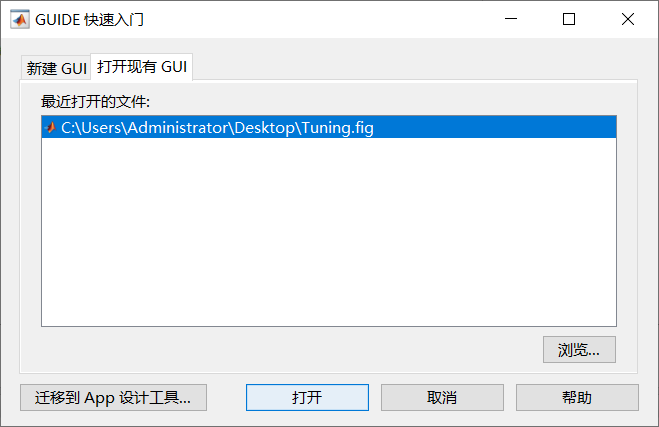打开设计好的GUI界面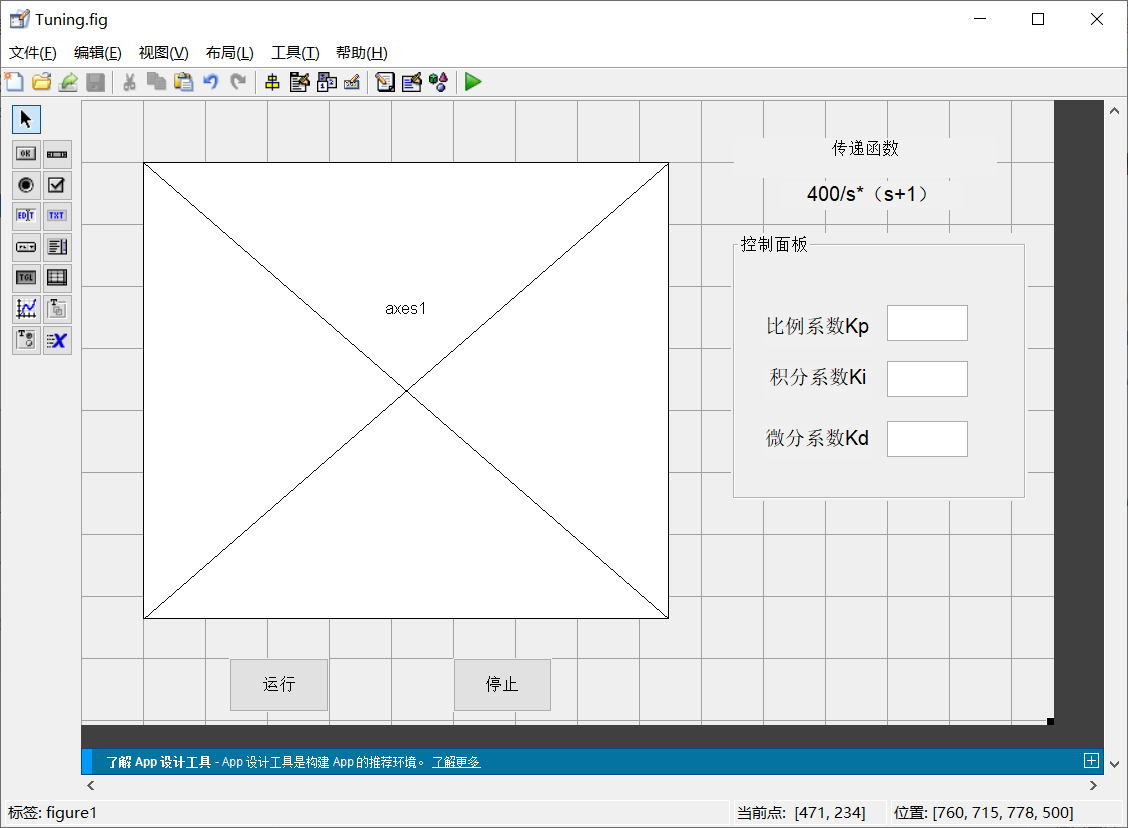在运行按钮的句柄下放置之前博客所介绍的----运用PID控制器优化传递函数的阶跃响应曲线。
function pushbutton1_Callback(hObject, eventdata, handles)
% hObject    handle to pushbutton1 (see GCBO)
% eventdata  reserved - to be defined in a future version of MATLAB
% handles    structure with handles and user data (see GUIDATA)
kp = get(handles.edit1,'String');  % 获取P值
ki = get(handles.edit2,'String');  % 获取I值
kd = get(handles.edit3,'String');  % 获取d值

KP=str2double(kp);  %因为edit默认都是字符，需要转为数值进行运算
KI=str2double(ki);
KD=str2double(kd);

% s=tf('s');
% PID=KP+KD*s+KI/s;  % 获得pid传递函数
% P = 1/(s^2 + 10*s + 20);
% T = feedback(PID*P,1);
% t = 0:0.01:2;
% y=step(T,t);  % 阶跃响应
% plot(t,y);   % 绘图

%% 定义传递函数
ts=0.001;
sys=tf(400,[1,1,0]); %% 400/（s（s+1））
dsys=c2d(sys,ts,'z');
[num,den]=tfdata(dsys,'v');

rin=1.0;
u_1=0.0;u_2=0.0;
y_1=0.0;y_2=0.0;
x=[0,0,0]';
error_1=0;
P=1000;

%% 定义PID控制参数
Kpidi(1) = KP;%0-30
Kpidi(2) = KI;%0-1
Kpidi(3) = KD;%0-1

for k=1:1:P
timef(k)=k*ts;
r(k)=rin;
u(k)=Kpidi(1)*x(1)+Kpidi(2)*x(2)+Kpidi(3)*x(3);

if u(k)>=10
u(k)=10;
end
if u(k)<=-10
u(k)=-10;
end

yout(k)=-den(2)*y_1-den(3)*y_2+num(2)*u_1+num(3)*u_2;
error(k)=r(k)-yout(k);

%------------ Return of PID parameters -------------
u_2=u_1;u_1=u(k);
y_2=y_1;y_1=yout(k);

x(1)=error(k);                % Calculating P
x(2)=(error(k)-error_1)/ts;   % Calculating D
x(3)=x(3)+error(k)*ts;        % Calculating I
error_2=error_1;
error_1=error(k);

end
plot(timef,rin,'r',timef,yout,'b');
xlabel('Time(s)');ylabel('rin,yout');
end

二、参数整定的运行结果展示
运行程序Tuning.m，未输入三个kp、ki和kd 输出的结果如下：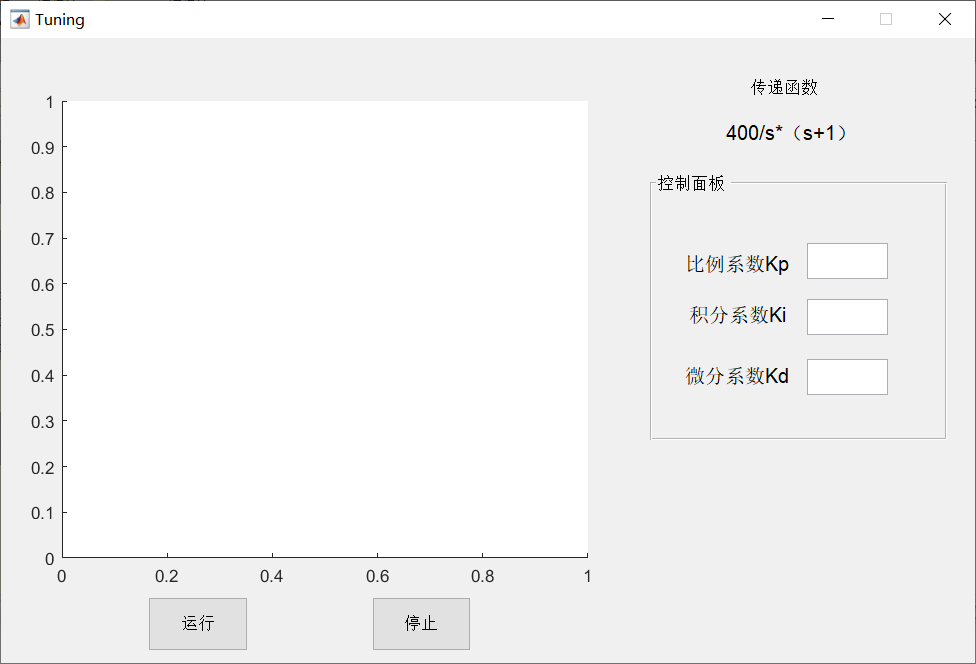最终调整后，所优化的结果如下：kp=28；ki=0.5；ki=0.01，结果如下；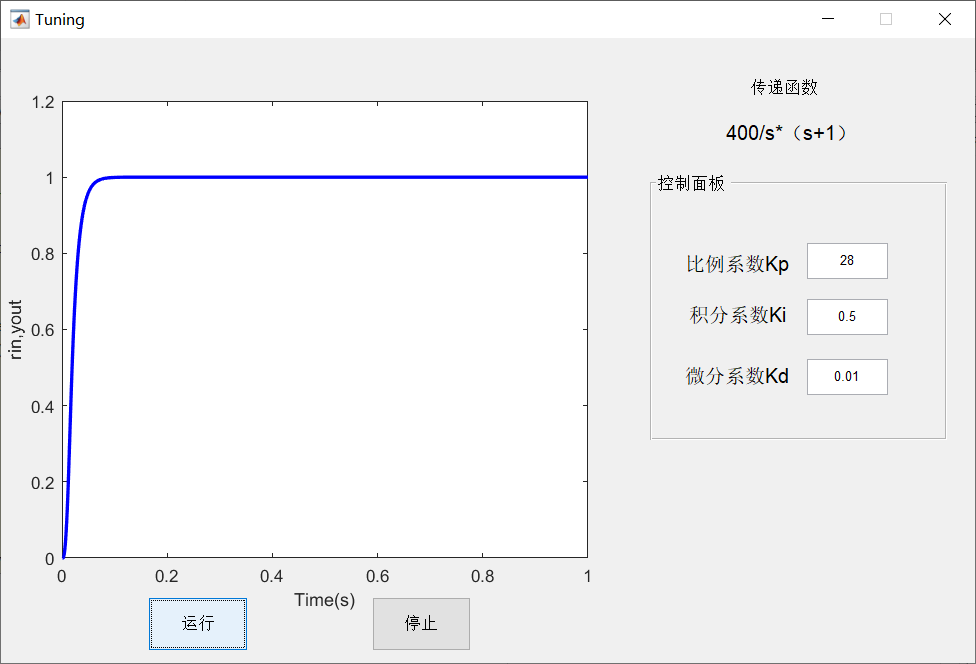1.Kp变化的结果
当kp=38；ki=0.5；ki=0.01，运行结果如下：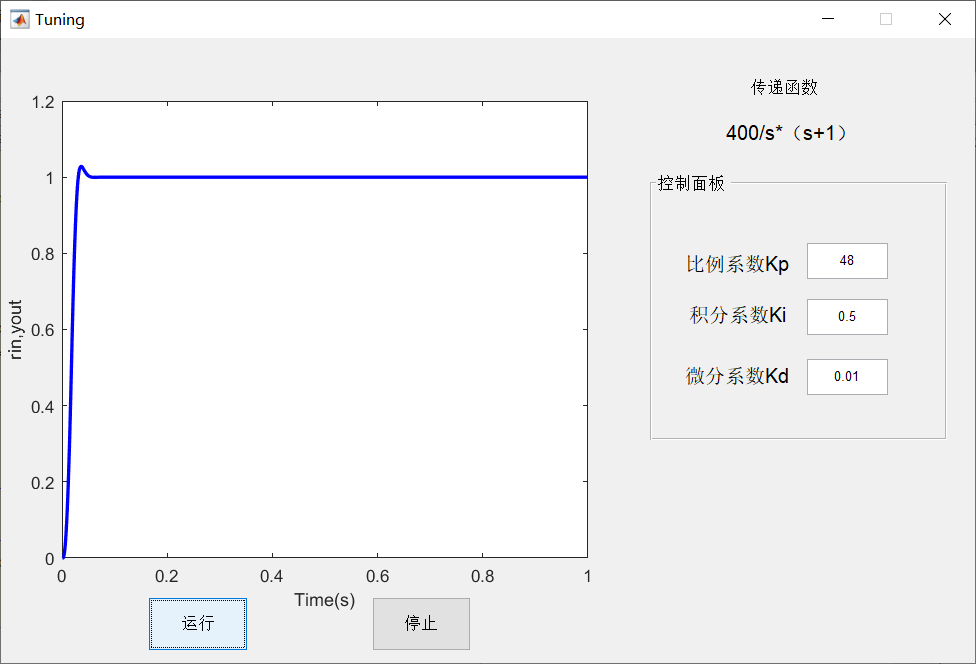当kp=8；ki=0.5；ki=0.01，运行结果如下：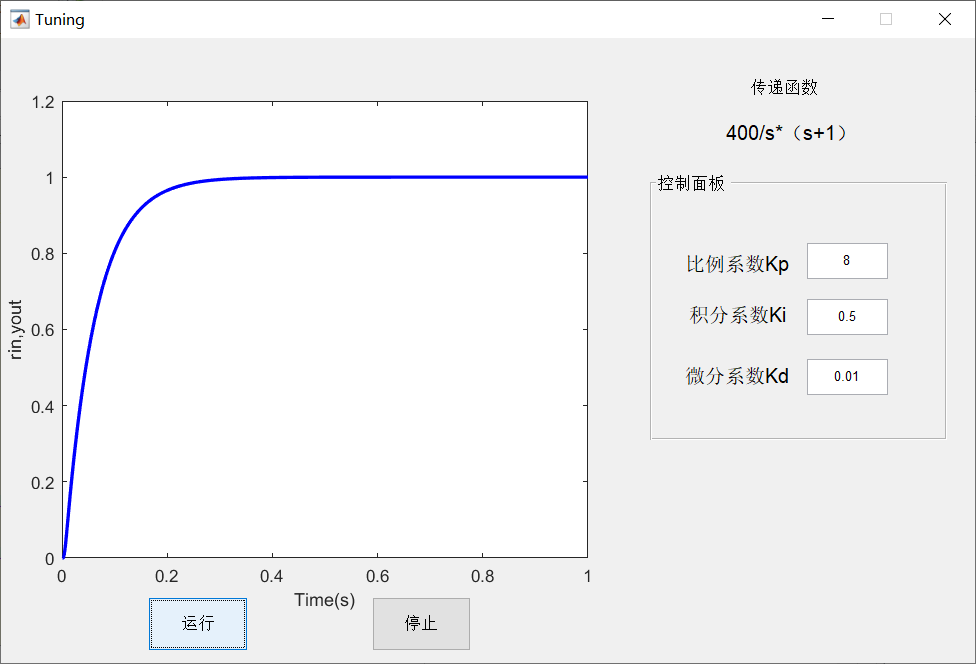由上可知，增大比例系数Kp会加快系统响应但会带来超调。
2.Ki变化的结果
当kp=28；ki=5；ki=0.01，运行结果如下：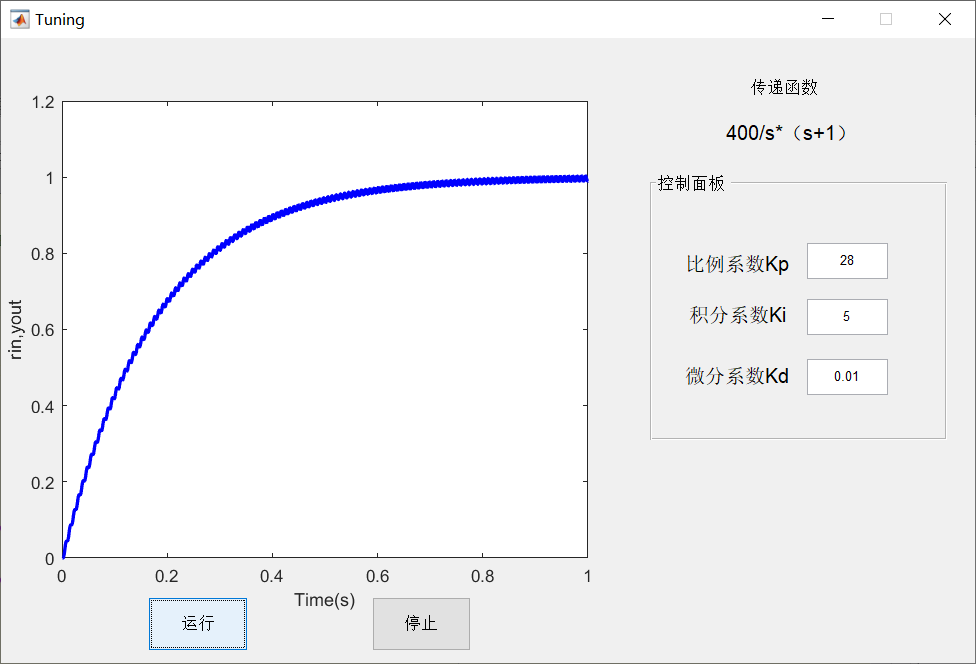当kp=28；ki=0.05；ki=0.01，运行结果如下：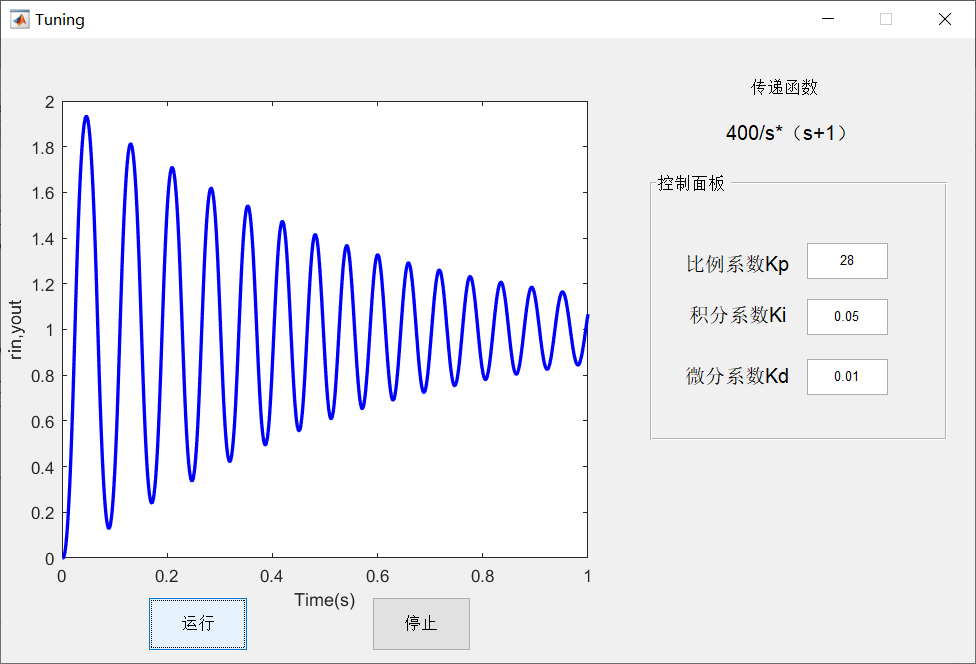由上可知，增大积分系数Ki有利于减小超调，减小振荡，但系统响应速度变慢。过小的Ki会导致系统不稳定。
3.Kd变化的结果
当kp=28；ki=0.5；ki=1，运行结果如下：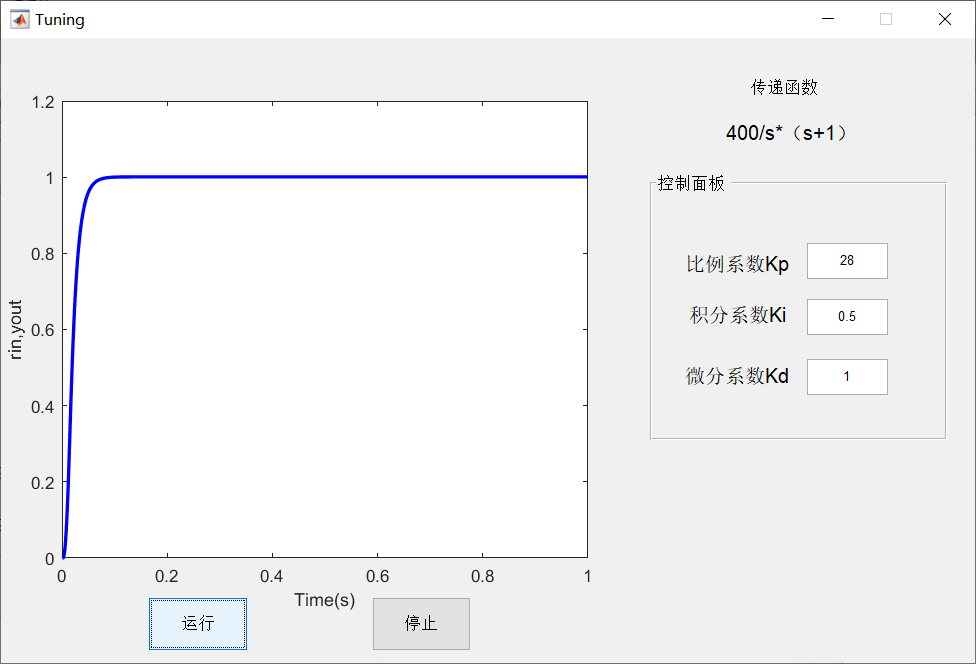当kp=28；ki=0.5；ki=0.0001，运行结果如下：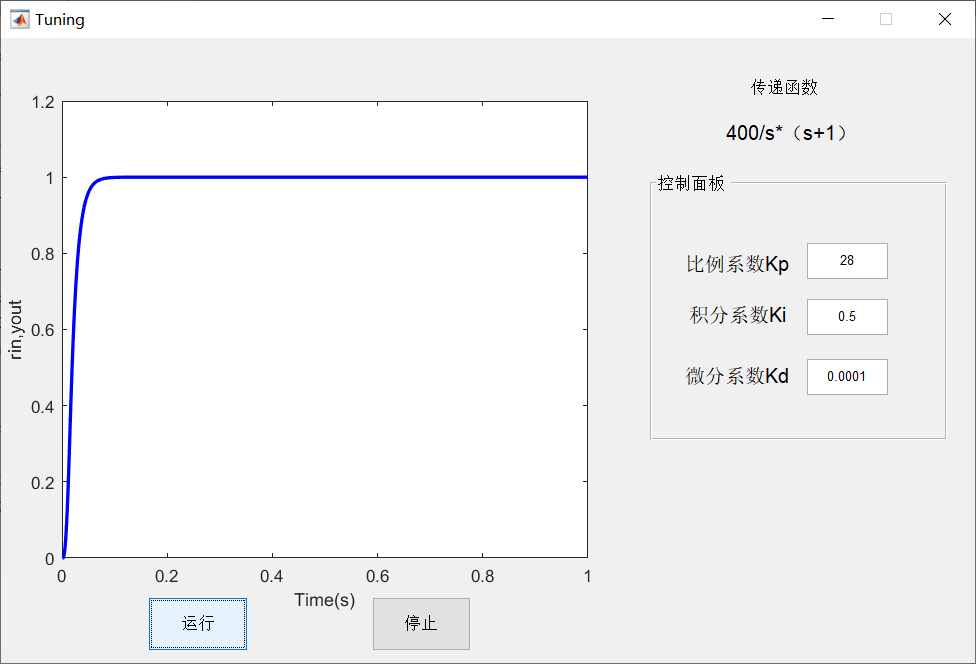由上可知，对于精确的1型2阶系统而言微分环节作用影响较小，主要是由于微分的作用是提高系统抗扰动能力，降低系统抗噪声能力。后续可以在联合仿真模型给予一定的扰动，再进行试验的验证。
总结

1.后续博客的研究是将前述所讲的算法在线整定PID参数，融合到这个Matlab/GUI界面设计上，可以实时观看参数变化、曲线变化以及适应度函数大小值变化的曲线图。
2.将这个思路拓展到QT平台上，运用控制板进行实际效果验证。


展开全文gui
• 仅作记录用途 matlab -nodesktop -nosplash ...matlab -nodisplay ...matlab -nojvm -nosplash ...matlab程序也可以在命令行里直接运行，只需要...比如运行当前目录下example.m matlab -nodesktop -nosplash -r
• :在 MATLAB 命令窗口(Command Window)中运行guide命令，来打开 GUIDE 界面，如下： >_<:然后，选择空模板(Blang GUI)，点击 OK，即可打开 GUIDE 设计界面，如下： >_<:点击工具栏上菜单编辑器...
• 在Linux系统中运行MATLAB程序时，假设要运行的MATLAB文件为test.m，如果直接使用matlab test就会默认跳出MATLAB图形界面进行可视化操作。因此我们要想办法通过命令不打卡MATLAB图形界面。 方法 还是假设要运行的...linux
• 1、在每台服务器上安装并行运行包，进入cd /opt/matlab/toolbox/distcomp/bin/ 执行：sudo ./mdce install ，新版建议执行：mjs install 2、并启动集群运行包 3、启动集群配置界面 4、先进行ip映射，在每个节点/...
• 去掉MATLAB编译产生可执行图形界面程序运行DOS黑窗口一种方法
• 本版在上一版的基础上修正了上版出的严重bug,并增加了服务器端的配置，使得在整个并行框架搭建的时候通过界面就可以进行配置并得到相应的运行信息。 还在用matlab编串行程序而几天几夜的跑不完么？为什么不试一下...
• ## Matlab简易GUI界面

千次阅读 2018-07-16 00:57:20
运行命令guide，打开GUI界面编辑器，通过左侧选项添加各种界面组件，如按钮、文本框等，双击组件弹出组件属性编辑框，可以定义按钮等文本内容、字号、颜色以及回调函数等。对于按钮点击所触发回调函数，双击按钮...GUI
• Matlab-GUI程序实现 界面实时显示命令行中运行过程 在Matlab的GUI程序开发中，我们常常先测试完成部分的代码构成一个函数脚本，将来以便在最终GUI开发时直接调用函数。 这种开发思路没有任何问题，也很高效，代码也...
• 首先参考命令行下matlab的运行参数的定义与作用：http://www.cnblogs.com/beanocean/p/3677404.html 创建示例程序：test.m [plain] view plain copy functiontest(x,y) fprintf(num2str(x+y)) ...
• 基于Matlab/GUI界面设计参数在线整定与优化（三） 基于Matlab/GUI界面设计参数在线整定与优化（二） 在这篇博客基础之上，将区间优化参数设定最大值与遗传算法设定值释放并给出客户端这边进行设置，具体...
• 基于Matlab/GUI界面设计参数在线整定与优化（二） 参考前述博客：基于Matlab/GUI界面设计参数在线整定与优化（一） 将之前运用GA算法整定博客：基于遗传算法PID参数整定研究（八） 1.界面显示 2.操作流程...
• Matlab app designer界面（二） 一.从excel到表数据读取 关于表这里提供了很多选择，可根据自己需求进行相应修改。 首先应在matlab里面找到表，不然会显示错误。我表叫book1.xlsx 第二步，设计视图 右击...
• 第1章 MATLAB操作基础 1.1 MATLAB概述 1.2 MATLAB的运行环境与安装 1.3 MATLAB集成环境 1.4 MATLAB帮助系统;1.1 MATLAB概述;1.1.2 MATLAB的主要功能 1数值计算和符号计算功能 MATLAB以矩阵作为数据操作的基本单位还...
• MATLAB GUI设计时，插入axes控件，在运行时，如何去除坐标轴和坐标刻度，并且保留窗口白色背景？ 例如： 运行效果： 期望得到效果：   这里提供两种解决办法： 第一种办法：（推荐，设置起来...axes控件
• 传说中的MATLAB1.0（1984年DOS界面182K、运行良好）附下载 你见过 MATLAB 最早版本吗？下载一份 MATLAB 1.0,(只有182K, 只有20来个函数) 体会一下吧。MATLAB早期表示矩阵是用 <> 而不是 []。而今日的matlab...
• MATLAB的命令窗口(Command Window)中运行guide命令，来打开GUIDE界面，如下： 然后，选择空模板(Blang GUI)，点击OK，即可打开GUIDE的设计界面，如下： 点击工具栏上的菜单编辑器(Menu Editor)，打开菜单编辑器，...GUI
• 图形用户界面(Graphical User Interface,GUI) 是指由窗口、菜单、光标、按键、对话框和文本等各种图形对象组成用户界面，用来定制用户与Matlab 交互方式（命令窗口不是用户唯一与Matlab 交互方式）。...gui
• matlab app运行界面切换出错问题及解决方案 matlab app编写 目前，使用matlab编写基于matlab环境app有两种方式，一种是传统GUI功能，还有一种是The MathWorks公司在MATLAB R2016a推出AppDesigner功能，后...app
• 本文为CSDN博主「米饭白色」原创文章 原文链接：... 1. 下面是我自己设置 可以将如下命令加到~/.bashrc文件 # ########## Matlab 2016b ########### #1. set matlab path PATH=......

# matlab的运行界面matlab 订阅